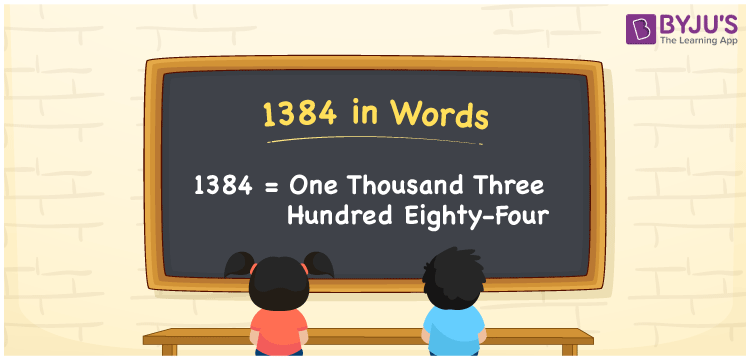# 1384 in Words

We can write 1384 in words as One thousand three hundred eighty-four. If you purchased a kg of specially made sweets for Rs. 1384, then you can say, “I purchased a kg of specially made sweets for One thousand three hundred eighty-four rupees”. Here, One thousand three hundred eighty-four is the word form of the cardinal number 1384.

 1384 in words One thousand three hundred eighty-four One thousand three hundred eighty-four in Numbers 1384

## 1384 in English Words

We generally write numbers in words using the English alphabet. So, we spell 1384 in words as “One thousand three hundred eighty-four”.## How to Write 1384 in Words?

In this section, you will learn how to write the number 1384 in words with the help of a place value chart given below.

 Thousands Hundreds Tens Ones 1 3 8 4

Here, ones = 4, tens = 8, hundreds = 3, and thousands = 1.

Let us expand the above digits based on their place value.

1 × Thousand + 3 × Hundred + 8 × Ten + 4 × One

= 1 × 1000 + 3 × 100 + 8 × 10 + 4 × 1

= 1000 + 300 + 80 + 4

= One thousand + Three hundred + Eighty + Four

= One thousand three hundred eighty-four

Therefore, 1384 in words = One thousand three hundred eighty-four.

1384 is a natural number that precedes 1385 and succeeds 1383.

1384 in words – One thousand three hundred eighty-four

Is 1384 an odd number? – No

Is 1384 an even number? – Yes

Is 1384 a prime number? – No

Is 1384 a composite number? – Yes

Is 1384 a perfect square number? – No

Is 1384 a perfect cube number? – No

## Frequently Asked Questions on 1384 in Words

Q1

### How do you write 1384 in words?

We can write the number 1384 in words as One thousand three hundred eighty-four.
Q2

### How to write 1384 rupees in words on a cheque?

On a cheque, we generally write 1384 rupees in words as “One thousand three hundred eighty-four rupees only”.
Q3

### Find the value of 1384 + 166.

1384 + 166 = 1550 Therefore, the value of 1384 + 166 is 1550.